# KSEEB SSLC Class 10 Maths Solutions Chapter 2 Triangles Ex 2.4

In this chapter, we provide KSEEB SSLC Class 10 Maths Solutions Chapter 2 Triangles Ex 2.4 for English medium students, Which will very helpful for every student in their exams. Students can download the latest KSEEB SSLC Class 10 Maths Solutions Chapter 2 Triangles Ex 2.4 pdf, free KSEEB SSLC Class 10 Maths Solutions Chapter 2 Triangles Ex 2.4 book pdf download. Now you will get step by step solution to each question.

### Karnataka State Syllabus Class 10 Maths Chapter 2 Triangles Ex 2.4

Question 1.
Let ∆ABC ~ ∆DEF and their areas be, respectively, 64 cm2 and 121 cm2, If EF = 15.4 cm., find BC.
Solution: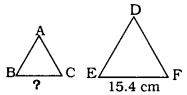If ∆ABC ~ ∆DEF, then
ar(ΔABC)ar(ΔDEF)=BC2EF2
64121=BC2(15.4)2
BC2=64121=BC2(15.4)2
BC2=64121×237.161
∴ BC = 8 × 1.4
∴ BC = 11.2 cm

Question 2.
Diagonals of a trapezium ABCD with AB || DC intersect each other at the point O. If AB = 2 CD, find the ratio of the areas of triangles AOB and COD.Solution:
Data: Diagonals of a trapezium ABCD with AB||DC intersect each other at the point O. We have AB = 2 CD.
To prove: Ar(ΔAOB)Ar(ΔCOD)=?
As per similarity criterion, ∆AOB and ∆COD are,
∆AOB ~ ∆COD
Ar(ΔAOB)Ar(ΔCOD)=AB2DC2
=(2DC)2DC2(⋅AB=2DC)
=4DC21DC2
Ar(ΔAOB)Ar(ΔCOD)=41
= 4 : 1

Question 3.
In the given figure, ABC and DBC are two triangles on the same base BC. If AD intersects BC at O, show that
Ar(ΔABC)Ar(ΔDBC)=AODO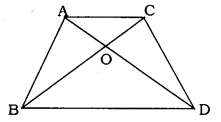Solution:
Data: ABC and DBC are two triangles on the same base BC. If AD intersects BC at O.
To Prove: Ar(ΔABC)Ar(ΔDBC)=AODO
Construction: Draw AP ⊥ BC and DM ⊥ BC.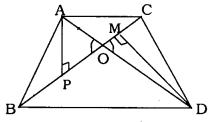Area of ∆ = 12 × base × height
Ar(ΔABC)Ar(ΔDBC)=12×BC×AP12×BC×DM
=APDM
In ∆APO and ∆DMO,
∠APO = ∠DMO = 90° (construction)
∠AOP = ∠DOM (Vertically opposite angles)
∴ ∆APO ~ ∆DMO
(∵ Similarity criterion is A.A.A.)
APDM=AODO
⇒Ar(ΔABC)Ar(ΔDBC)=AODO

Question 4.
If the areas of two similar triangles are equal, prove that they are congruent.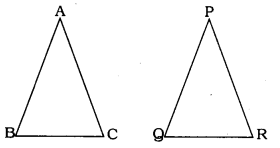Solution:
In ∆ABC ~ ∆PQR
Ar(ΔABC)Ar(ΔPQR)=(ABPQ)2=(BCQR)2=(ACPR)2 …………. (1)
Area of ∆ABC = Area of ∆PQR
⇒Ar(ΔABC)Ar(ΔPQR)=1 …………. (2)
Substituting the eqn (2) in eqn (1)
1=(ABPQ)2=(BCQR)2=(ACPR)2
⇒ AB = PQ, BC = QR, AC = PR
∴ ∆ABC ≅ ∆PQR (∵ S.S.S postulate)

Question 5.
D, E, and F are respectively mid-points of sides AB, BC and CA of ∆ABC. Find the ratio of the areas of ∆DEF and ∆ABC.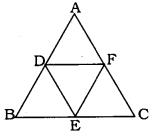Solution:
Data: D. E and F are respectively midpoints of sides AB, BC and CA of ∆ABC.
To Prove: Ratio of the areas of ∆DEF and ∆ABC.
If a line divides two sides of a triangle in the same ratio, then the line is parallel to the third side and it is half of that side
DE∥ACDE=12AC
EF∥ABEF=12AB
DF∥BCDF=12BC
Ar(ΔDEF)Ar(ΔABC)=DF2BC2(Theorem6)
Ar(ΔDEF)Ar(ΔABC)=(12BC)2BC2
Ar(ΔDEF)Ar(ΔABC)=14BC2BC1=14=1:4

Question 6.
Prove that the ratio of the areas , of two similar triangles is equal to the square of the ratio of their corresponding medians.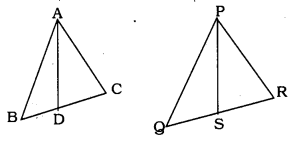Solution:
Let ∆ABC ~ ∆PQR
AD and PS are mid-point lines of ∆ABC and ∆PQR.
∆ABC ~ ∆PQR
ABPQ=BCQR=ACPR ………………… (1)
∠A = ∠P, ∠R = ∠Q, and ∠C = ∠R (2)
AD and PS are mid-point lines
BD=DC=BC2
and QS=SR=QR2
ABPQ=BDQS=ACPR …………… (3)
In ∆ABD and ∆PQS,
∠B = ∠Q ………. Eqn (2)
and ABPQ=BDQS ………. Eqn (3)
∴ ∆ABD ~ ∆PQS (SAS Postulate)
Ar(ΔABC)Ar(ΔPQR)=(ABPQ)2=(BCQR)2=(ACPR)2
From Eqn. (1) and Eqn. (4), we have

Question 7.
Prove that the area of an equilateral triangle described on one side of a square is equal to half the area of the equilateral triangle described on one of its diagonals.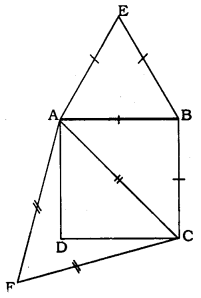Solution:
Data: Equilateral triangle ABE is on AB of square ABCD. Equilateral triangle ACF is on diagonal AC.
To Prove: (ΔABE)=12 Ar (ΔACF)
Ar(ΔABE)Ar(ΔACF)=AB2AC2Theorem6
=AB2AB2+BC2
∵ Pythagoras theorem
=AB22AB2
∴ AB = AC sides of square
=1×AB22×AB2
Ar(ΔABE)Ar(ΔACF)=12

Tick the correct answer and justify:
Question 8.
∆ABC and ∆BDE are two equilateral triangles such that D is the mid-point of BC. The ratio of the areas of triangles ∆ABC and ∆BDE is :
A) 2 : 1
B) 1 : 2
C) 4 : 1
D) 1 : 4
Solution:
C) 4 : 1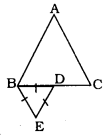Ar(ΔABC)Ar(ΔBDE)=BC2BD2=BC2(12BC)2
=11/4=41
= 4 : 1

Question 9.
Sides of two similar triangles are in the ratio 4 : 9. Areas of these triangles are in the ratio :
A) 2 : 3
B) 4 : 9
C) 81 : 16
D) 16 : 81
Solution:
D) 16 : 81
∴ (4)2 : (9)2 = 16 : 81.

All Chapter KSEEB Solutions For Class 10 Maths

—————————————————————————–

All Subject KSEEB Solutions For Class 10

*************************************************

I think you got complete solutions for this chapter. If You have any queries regarding this chapter, please comment on the below section our subject teacher will answer you. We tried our best to give complete solutions so you got good marks in your exam.

If these solutions have helped you, you can also share kseebsolutionsfor.com to your friends.

Best of Luck!!